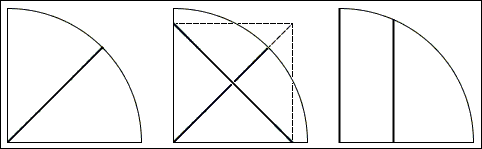## Shortest Fence in a Quarter-Circle Pasture

Here is the problem:

 A farmer needs to divide equally a quarter-circle pasture between two of his cows. He seeks to build a shortest straight line fence. Which of the three ways below should he choose?Solution## Solution

A solution transpires from the following diagram:In the first variant, the fence is built along a radius of the circle and is equal the radius in length. In a square, like in any rectangle, diagonals are equal. Therefore, in the second variant the fence is longer than the radius. In the third variant, the fence is clearly shorter than the radius. This is the way to go.

## References

1. R. Blum et al., Mathemagic, Main Street, 2002